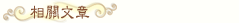# 【未解之謎】用數學公式證明神的存在【字號】
標籤: , , , ,

## 大洋彼岸的神祕來信

1913年的一個冬日，英國劍橋大學三一學院的數學教授哈代在吃完早飯之後，按照慣例拿起桌邊的《泰晤士》報紙，準備了解當日新聞。這時，報紙上放著的一封信引起了他的注意。信封上貼著來自印度的郵票，並寫著「馬德拉斯，1913年1月16日」。雖然自己似乎在印度馬德拉斯沒有熟人，哈代還是打開了信封，抽出了長達10頁的信。

## 天才的鼎盛時期

2=2, 2=1+1, p(2)=2
3=3, 3=1+1+1, 3=1+2, p(3)=3
4=4, 4=1+1+1+1, 4=1+1+2, 4=1+3, 4=2+2, p(4)=5
……..

## 最後的時刻